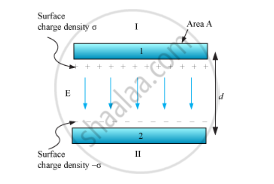# If Two Similar Large Plates, Each of Area a Having Surface Charge Densities +σ and –σ Are Separated by a Distance D in Air, Find the Expressions - Physics

(i) If two similar large plates, each of area A having surface charge densities +σ and –σ are separated by a distance d in air, find the expressions for

(a) field at points between the two plates and on outer side of the plates. Specify the direction of the field in each case.

(b) the potential difference between the plates.

(c) the capacitance of the capacitor so formed.

(ii) Two metallic spheres of Radii R and 2R are charged so that both of these have same surface charge density σ. If they are connected to each other with a conducting wire, inn which direction will the charge flow and why?

#### Solution

(a)

We are given two similar large plates separated by a small distance (d) and having area (A).Surface charge density of plate 1, σ = Q/A, and that of plate 2 is − σ.

Electric field in different regions:

Outer region I,

E=sigma/(2epsilon_0)-sigma/(2epsilon_0)=0

Outer region II,

E=sigma/(2epsilon_0)-sigma/(2epsilon_0)=0

In the inner region between plates 1 and 2, the electric fields due to the two charged plates add up. So,

E=sigma/(2epsilon_0)+sigma/(2epsilon_0)=sigma/epsilon_0=Q/(epsilon_0A)

The direction of electric field is from the positive to the negative plate.

(b)

For uniform electric field, potential difference is simply the electric field multiplied by the distance between the plates, i.e.

V=Ed=1/epsilon_0 (Qd)/A

(c)

Capacitance of the parallel plate capacitor,

C=Q/V=(epsilon_0A)/d

(ii)

We know that the potential of the metallic sphere is given by,

V=Q/(4piepsilon_0r)

Now, the potential of the metallic sphere of radius R is given by,

V_R=Q/(4piepsilon_0R)

V_R=(sigma(4piR^2))/(4piepsilon_0R)

V_R=(sigmaR)/epsilon_0

Similarly, the potential of the metallic sphere of radius 2R is given b

V_(2R)=Q/(4piepsilon_0 2R)

V_(2R)=(sigma(4pi(2R)^2))/(4piepsilon_0 2R)

V_(2R)=(sigma2R)/epsilon_0

from the relation (i) and (ii) we know that V2R VR

So, when both the spheres are connected the charge flow from the sphere of radius of 2R to R.

Concept: Electric Field - Introduction of Electric Field
Is there an error in this question or solution?